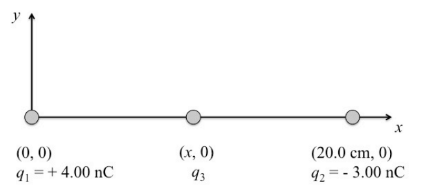Problem: A point charge q1 = 4.00 nC is placed at the origin. A second point charge q 2 = -3.00 nC is placed on the x-axis at x = 20.0 cm. A third point charge q3 = 2.00 nC is placed on the x-axis between q1 and q2. Assume that the potential energy of the three charges is zero when they are infinitely far apart. Determine the electric potential energy of the system of the three charges  if q3 is placed at x = 10.0 cm.

92% (3 ratings)
Problem Details

A point charge q1 = 4.00 nC is placed at the origin. A second point charge q 2 = -3.00 nC is placed on the x-axis at x = 20.0 cm. A third point charge q3 = 2.00 nC is placed on the x-axis between q1 and q2. Assume that the potential energy of the three charges is zero when they are infinitely far apart. Determine the electric potential energy of the system of the three charges  if q3 is placed at x = 10.0 cm.